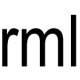# Reverse the words in a sentence – C++ solution and test code

Here is a solution to the standard interview questions of reversing the letters in the words of a sentence. It is a more complex version of the even more common “reverse a string” question. I also include my test code.

There are two main parts to this. The first identifies where the word boundaries are. The second reverses the letters between two positions in the array.

The wordStart and wordEnd variables keep track of the word boundaries. We first look for the start of the word and then the end and store the positions. These values are then passed to ReverseWord along with the character array. ReverseWord does some simple character swapping to reverse the word.

```void ReverseWords(char string[])
{
int length = StringLength(string);
int wordStart = -1;
int wordEnd = -1;
for (int i=0; i<length; ++i)
{
if (string[i] == ' ' && wordStart == -1)
{
continue;
}
else if (wordStart == -1)
{
wordStart = i;
}
if (wordStart != -1 && string[i] == ' ')
{
wordEnd = i-1;
ReverseWord(string, wordStart, wordEnd);
wordStart = -1;
wordEnd = -1;
}
}
if (wordStart != -1)
{
wordEnd = length-1;
ReverseWord(string, wordStart, wordEnd);
}
}

void ReverseWord(char string[], int wordStart, int wordEnd)
{
int midPoint = (wordStart+wordEnd)/2;
for (int l=wordStart, r=wordEnd; l<=midPoint; ++l, --r)
{
char tmp = string[l];
string[l] = string[r];
string[r] = tmp;
}
}
```

Here is the test code. For each test a character array is passed to the word reversing function. The result is then compared against the expected result. If they match the test passes. If they don’t match then the test fails. I’ve tried to test the obvious cases. You could easily find flaws in the word reversal function but it is probably good enough to get you onto the next question.

```void ReverseWordsTest()
{
char test1[] = "cat and dog";
char expected1[] = "tac dna god";
ReverseAndCheck(test1, expected1);

char test2[] = "cat and dog ";
char expected2[] = "tac dna god ";
ReverseAndCheck(test2, expected2);

char test3[] = " cat and dog";
char expected3[] = " tac dna god";
ReverseAndCheck(test3, expected3);

char test4[] = " cat and dog ";
char expected4[] = " tac dna god ";
ReverseAndCheck(test4, expected4);

char test5[] = "cat  and dog";
char expected5[] = "tac  dna god";
ReverseAndCheck(test5, expected5);

char test6[] = "catanddog";
char expected6[] = "goddnatac";
ReverseAndCheck(test6, expected6);

char test7[] = "";
char expected7[] = "";
ReverseAndCheck(test7, expected7);
}

void ReverseAndCheck(char string[], char expected[])
{
ReverseWords(string);
if (MatchingStrings(string, expected))
{
cout << " Pass: " << string << endl;
}
else
{
cout << "!Fail: " << string << " != " << expected << endl;
}
}

bool MatchingStrings(char string1[], char string2[])
{
int length = StringLength(string1);
if (length != StringLength(string2))
{
return false;
}

for (int i=0; i<length; ++i)
{
if (string1[i] != string2[i])
{
return false;
}
}

return true;
}

int StringLength(char string[])
{
int index = 0;
char s = string[index];
while (s)
{
++index;
s = string[index];
}
return index;
}
```

### 2 Comments on “Reverse the words in a sentence – C++ solution and test code”

1.Brook Monroe says:

Is there some requirement that you avoid strtok or strpbrk, either of which would have made this trivial?

2.reviewmylife says:

Hi Brook, Often when interviewers ask a question like this they want you do do the solution using arrays rather than using higher level functions – as that would be too easy!

However if you are ever asked a question like this, it is certainly worth asking if you can use the higher level function if you know them. You might get credit for your knowledge of these APIs, even if they then tell you to solve it without using those functions.